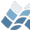NV5 GEOSPATIAL SOFTWAREDOTP

DOTP

## Purpose

Calculates the scalar product of two vectors.

Math

## Calling Sequence

Result = DOTP(A, B)

## Inputs

A: First vector.
B: Second vector.

## Outputs

The scalar product of A and B.

## Example

a = [1., -1.5, 2.]
b = [-3., -2., 1.5]